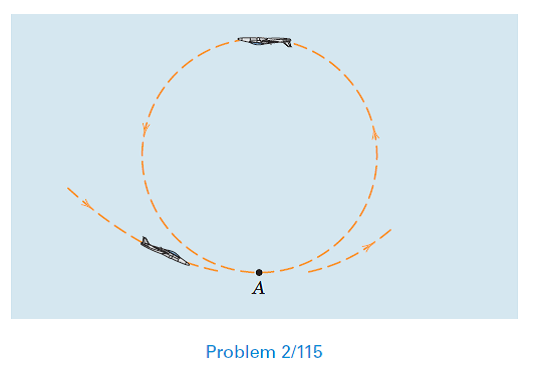Problem

# At the bottom A of the vertical inside loop, the magnitude of the total acceleration of...

At the bottom A of the vertical inside loop, the magnitude of the total acceleration of the airplane is 3g. If the airspeed is 800 km / h and is increasing at the rate of 20 km / h per second, calculate the radius of curvature P of the path at A.#### Step-by-Step Solution

Solution 1

Calculate the total acceleration.

The acceleration of the airplane is given below.

$$a_{n}=3 g$$

Substitute $$9.81 \mathrm{~m} / \mathrm{s}$$ for $$g$$.

\begin{aligned} a &=3 \times 9.81 \mathrm{~m} / \mathrm{s} \\ &=29.43 \mathrm{~m} / \mathrm{s} \end{aligned}

Calculate the rate of increase of air speed $$a_{t}$$.

\begin{aligned} a_{t} &=\frac{20 \times 1000 \times\left(\frac{\mathrm{m}}{\mathrm{s}}\right)}{3600(\mathrm{~s})} \\ &=5.55 \mathrm{~m} / \mathrm{s}^{2} \end{aligned}

Convert the units of velocity in to $$\mathrm{m} / \mathrm{s}$$.

$$v=800 \mathrm{~km} / \mathrm{h}$$

$$=\frac{800 \times 1000}{3600}$$

$$=222.22 \mathrm{~m} / \mathrm{s}$$

\begin{aligned} a^{2} &=a_{n}^{2}+a_{t}^{2} \\ &=\left(\frac{v^{2}}{\rho}\right)+a_{t}^{2} \end{aligned}

Here, $$a_{n}$$ and $$a_{t}$$ are the normal and tangential accelerations and $$v$$ is the velocity and $$\rho$$ is the radius of curvature.

Substitute $$29.43 \mathrm{~m} / \mathrm{s}^{2}$$ for $$a, 5.55 \mathrm{~m} / \mathrm{s}^{2}$$ for $$a_{t}$$ and $$222.22 \mathrm{~m} / \mathrm{s}$$ for $$v$$.

\begin{aligned} 29.43^{2} &=\left(\frac{222.22^{2}}{\rho}\right)^{2}+5.55^{2} \\ 29.43^{2}-5.55^{2} &=\left(\frac{222.22^{2}}{\rho}\right)^{2} \\ 835.322 &=\left(\frac{222.22^{2}}{\rho}\right)^{2} \\ \frac{835.322}{222.22^{4}} &=\frac{1}{\rho^{2}} \\ \rho=1708.6 \mathrm{~m} & \end{aligned}

Therefore, the radius of curvature $$\rho$$ is $$1708.6 \mathrm{~m}$$.